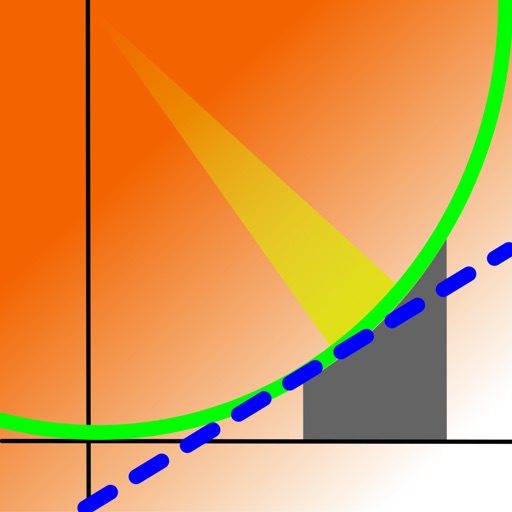## V-Graph Save is a scientific graphing calculator for iPhone and iPad that is designed to solve real problems in engineering and science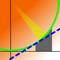# V-Graph Save

by Staghorn Ridge Software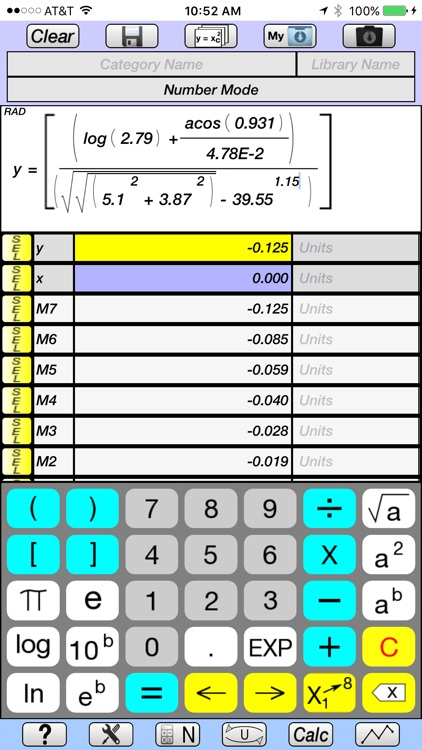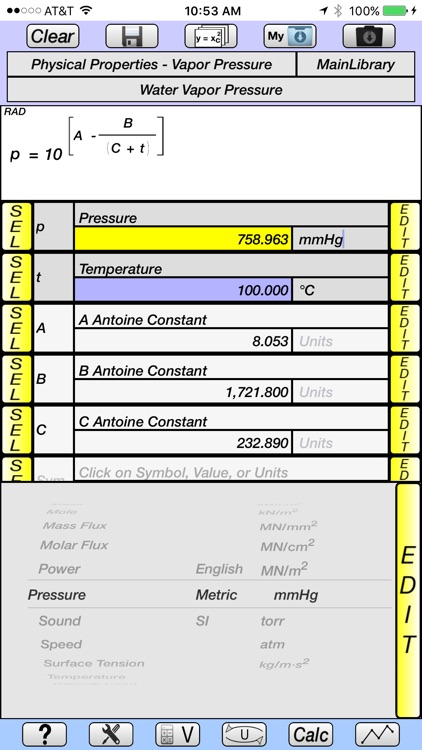V-Graph Save is a scientific graphing calculator for iPhone and iPad that is designed to solve real problems in engineering and science.### App Details

Version
1.4
Rating
(2)
Size
10Mb
Genre
Utilities Productivity
Last updated
January 31, 2021
Release date
January 17, 2017

### App Screenshots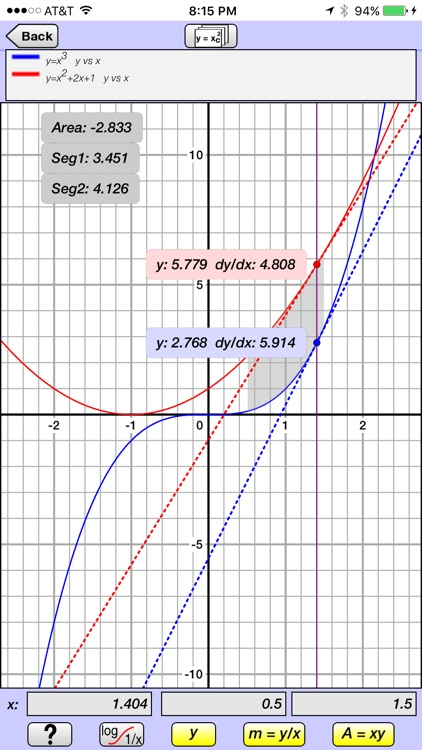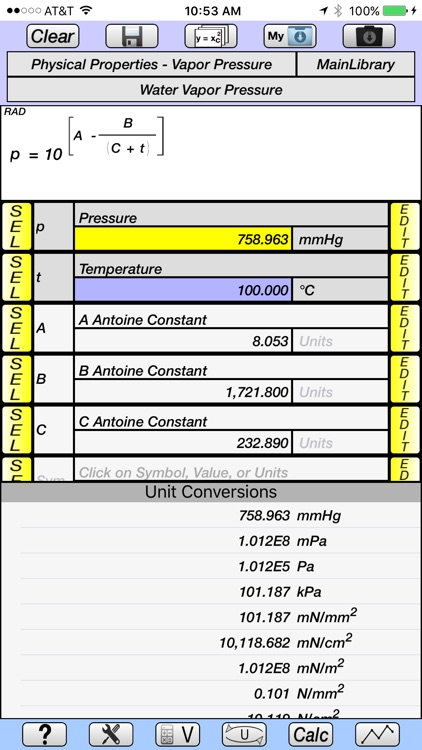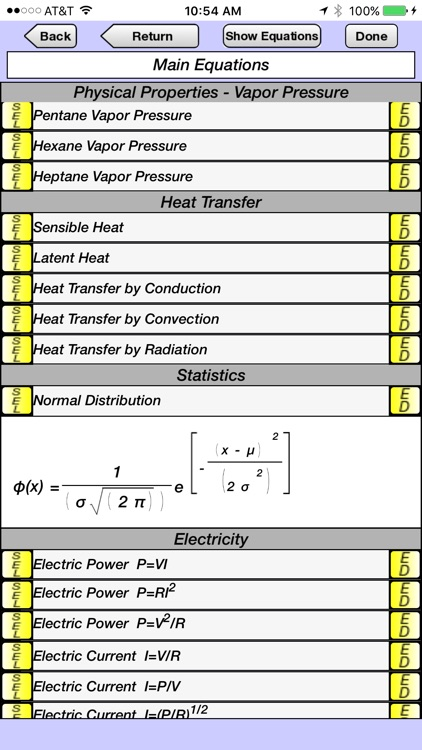### App Store Description

V-Graph Save is a scientific graphing calculator for iPhone and iPad that is designed to solve real problems in engineering and science.

(V-Solve is a free app that has all of the features of V-Graph Save except for graphing and being able to save equations to the library. V-Solve has a demo feature which shows the graphing features of V-Graph Save for a select set of equations.)

Overview of Features

• Solves and plots explicit and implicit equations.
• Equations are entered and displayed in their natural form, as they would appear in a textbook.
• 59 categories of units in library, divided into english, metric, and SI systems.
• Equations, constants, and user-defined unit conversions can be saved into libraries.
• Well-organized Main, Trigonometric, English, and Greek keypads.
• Help screen overlay provides pop-up description of the function of key buttons.
• Tutorials provide demonstration of the calculator's capabilities by programmatically stepping through graphing, equation creation and saving to the library, and unit conversion examples.

Graphing

• Plots explicit and implicit equations.
• Up to 3 equations can be plotted simultaneously.
• Pinch and Pan capabilities.
• Linear, log, inverse, inverse for celsius, inverse for fahrenheit scales for both x and y axes.
• Cursor displays both the y-value and slope for each equation.
• Cursor can be controlled by a finger or by entering an exact x-value.
• Area under the curve and line segment length can be displayed for a specified x-value range for one equation.
• For two equations, the area between the curves and the line segment length for both curves are displayed.

Equation Creation and Display

• Equations are created and displayed in their natural form, as they would appear in a textbook.
• Number Mode allows equations based on y (dependent variable) and x (independent variable) to be quickly created, solved, plotted, and have unit conversions carried out on the dependent variable.
• Variable Mode allows equations with more complex variables to be created and saved to the equation library under user-defined categories.
• Up to 3 equations from the library can be plotted simultaneously.
• Super- and sub-scripting of variables.
• Functions include square root, squared, x to any power, log, ln, 10 to any power, e to any power, factorial, trigonometric (including inverse), hyperbolic (including inverse).
• Button selector allows the variable symbol or its value to be displayed in the equation.
• Previously used equations are maintained in memory and can be easily accessed.

Unit Conversions

• Unit conversions are made simultaneously, and are displayed as a table of all units of the same category.
• New units can be created and saved to the library, using the base units (m, s, g, ft, lb, etc).
• New units are easily created using picker dials for the metric prefix (k, M, G, etc), base units, and power of 1, 2, 3, etc.

Equation and Constant Libraries

• Equation library with categories of Algebra, Geometry, Physical Properties (Vapor Pressure), Heat Transfer, Statistics, and Electricity.
• Physics constant library.
• New Equations and Constants can be saved to libraries under user-defined categories.
• Equation library has a summary page for each equation, which includes a display of the equation, its variables in table format, and a detailed description.

Other Features

• E-mail contact.
• Memory stack maintains values, variable symbols, and equation names of an unlimited number of previous calculations.
• Multiple answers for implicit equations are saved to the memory stack.
• Period or comma can be used as decimal separator.
• Degrees or radians for trigonometric functions.
• Decimal or Scientific Notation, and number of significant digits can be easily specified.
• Convergence criteria and maximum number of iterations can be specified for implicit equations.

Disclaimer:
AppAdvice does not own this application and only provides images and links contained in the iTunes Search API, to help our users find the best apps to download. If you are the developer of this app and would like your information removed, please send a request to [email protected] and your information will be removed.